Search IntMath
Close

450+ Math Lessons written by Math Professors and Teachers

5 Million+ Students Helped Each Year

1200+ Articles Written by Math Educators and Enthusiasts

Simplifying and Teaching Math for Over 23 Years

# Spiral around a hyperboloid - the Effekt bridge

By Murray Bourne, 26 Aug 2018

I was impressed by the design of the Effekt bridge in Denmark. The bridge consists of a spiral around a hyperboloid, allowing visitors to enjoy 360° treetop views.

Our challenge is to model the spiral shape of the bridge. That is, we seek to find the equation representing this spiral.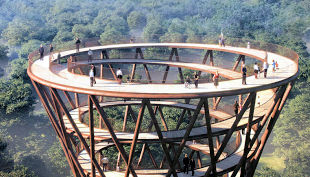EFFEKT bridge by Danish architects [source]

A hyperboloid is the 3-D object formed when we rotate a hyperbola around its axis. (A hyperbola is one of the conic sections, as are circles, ellipses and parabolas.)

Despite appearing top-heavy, hyperboloids are very strong structures since they are a doubly ruled surface (which means it can be supported by interlaced straight beams from the ground right up to the top of the structure.)

The dimensions of the hyperboloid on which our bridge is based is very close to 30 m diameter at the base, 15 m for the "skirt" diameter, and the structure is around 50 m high, as follows:EFFEKT bridge dimensions

The bridge involves 12 spirals up the side of a hyperboloid structure, as shown in this schematic: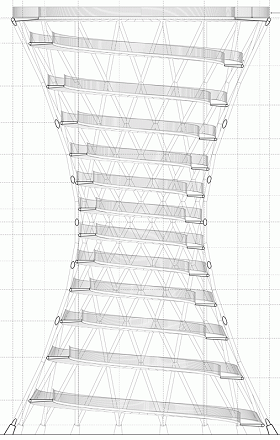Original bridge design [source]

What we're trying to do is to find a formula that will produce the above spiral, and will look something like the following: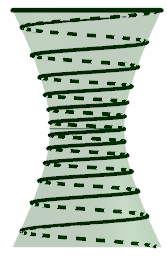Spiral bridge model

## The hyperboloid

First, we aim to draw the hyperboloid. It is not really necessary for this exercise, but it's good to be able to check that any spiral we find actually does hug the sides of the 3-D shape.

In general, the parametric equations for a hyperboloid with circular base are given by:

x = a cosh(v) cos(θ)

y = a cosh(v) sin(θ)

z = c sinh(v)

where

variable a affects how wide the hyperboloid is;

variable c affects the shape of the hyperboloid (higher c means the shape becomes more cylindrical); and

cosh(v) and sinh(v) are hyperbolic functions, defined by: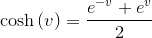and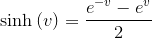The cos(θ) and sin(θ) are required to rotate the surface around the axis. (θ therefore takes values from 0 to 2π.)

We want the middle of the hyperboloid to have a radius of 7.5 m, so we choose a = 7.5. Now we need to determine the value of c.

At the base (and top) of the hyperboloid-shaped bridge, we need the value of x (and y) to have value 15. So we need to solve 7.5 cosh(v) = 15, to find the value of v there.

Solving such hyperbolic function equations requires numeric methods, so we use Wolfram|Alpha, which tells us if

cosh(v) = 15/7.5 = 2,

then

v = 1.32.

Now to find c. We need to substitute v = 1.32 and solve 25 = c sinh(1.32)

Once again, using Wolfram|Alpha we get c = 14.38.

So our parametric equations for the hyperboloid are:

x = 7.5 cosh(v) cos(θ)

y = 7.5 cosh(v) sin(θ)

z = 14.4 sinh(v)

where −1.32 ≤ v ≤ 1.32 and 0 ≤ θ ≤ 2π

## The spiral

The formula for the spiral is based on the cosh(v) and sinh(v) terms in the above expressions. However, we need to consider we have one less variable to play with. That is, we won't have access to the θ variable (since it's what we use to construct the surface, and won't produce the desired curve.)

So what we end up with is going to be in this form, using one main variable, u:

x = 7.5 cosh(mu) cos(u)

y = 7.5 cosh(mu) sin(u)

z = d sinh(nu)

The value 7.5 is the same as the hyperboloid case (since we need the "waist" to have a 7.5 m radius) and the cos(u) and sin(u) terms are necessary to make the spiral curve its way around the outside of the hyperboloid.

We need to determine the d, m and n values so that we'll have the correct spiral shape, and the correct number of spirals.

Since we need 12 spirals, the curve will need to wind around the shape 12 × 2π = 24π times. So we will have:

−12π ≤ u ≤ 12π

We only want the value inside the cosh function to go from −1.32to 1.32 (so the height of the spiral is 50 m), that is

−1.32 ≤ mu ≤ 1.32

So we need m = 1.32/12π = 0.035

At the top of the spiral, the z-value for the curve needs to be 25, and the x- and y-values need to be 15.

Since u = 12π at the top ofthe bridge, we have:

25 = d sinh (n ×12π)

This is one equation in two unknowns. Normally we can't solve such an equation, however we can choose a suitable pair of values for d and n as follows.

Let d = 7.5, (chosen for no other reason than that's the value used for the other 2 expressions, and is a reasonable first guess). Then solving the following equation using Wolfram|Alpha:

25 = 7.5 sinh (n ×12π)

gives us:

n = 0.051

Putting it all together, the parametric equations for our spiral are given by:

x = 7.5 cosh(0.035u) cos(u)

y = 7.5 cosh(0.035u) sin(u)

z = 7.5 sinh(0.051u)

Here's our model shown on the x-, y- and z-axes, after adding 25 to each of the z-expressions, placing the base on the x-y plane: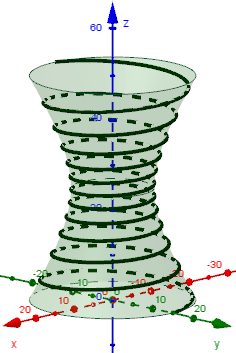Hyperboloid spiral with base on x-y plane.

## Is it correct?

Let's now check our hyperboloid and our spiral to see if we have done it correctly.

Here is my solution overlaid on the original schematic of the bridge.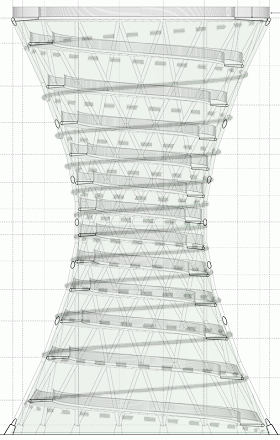Model overlaid on original bridge plans

It's close, but some of the path levels are a bit higher than my model, and some are a bit lower. This could be due to engineering requirements, for example placement of the supporting beams that may have conflicted with the "perfect" spiral that I have modelled.

## Conclusion

Our model for the spiral around a hyperboloid, approximating the EFFEKT tree top bridge in Denmark, seems quite reasonable. It is an interesting real-world application of mathematics.

The above graphics were obtained using Geogebra, an invaluable tool for this kind of task. I used several sliders for the variables involved so I could better check what effect each variable was having on the final outcome.

Be the first to comment below.

### Comment Preview

HTML: You can use simple tags like <b>, <a href="...">, etc.

To enter math, you can can either:

1. Use simple calculator-like input in the following format (surround your math in backticks, or qq on tablet or phone):
a^2 = sqrt(b^2 + c^2)
(See more on ASCIIMath syntax); or
2. Use simple LaTeX in the following format. Surround your math with $$ and $$.
$$\int g dx = \sqrt{\frac{a}{b}}$$
(This is standard simple LaTeX.)

NOTE: You can mix both types of math entry in your comment.